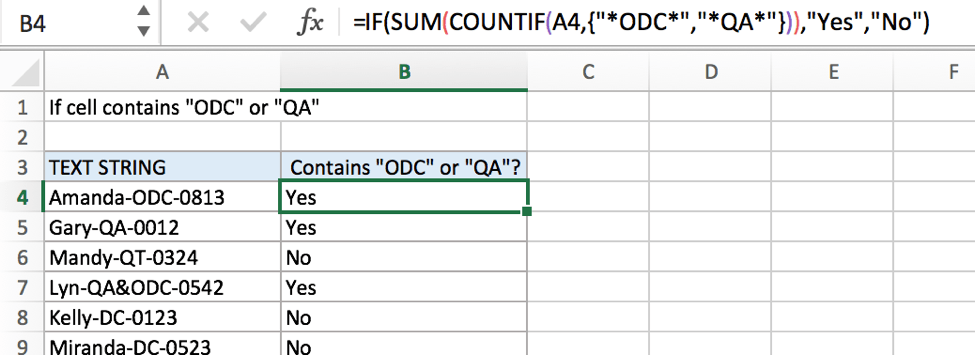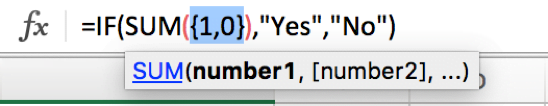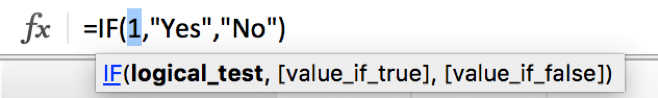# If Cell Contains Either Text1 or Text2

IF function is frequently used in Excel worksheet to return “true value” or “false value” based on the logical test result. If you want to see if cell contains certain substring1 like “abc” or substring2 like “def”, and returns true of false based on test result, you can build a formula based on IF function and together with SUM function and COUNTIF function.FORMULA

To test if cell contains certain text1 or text2, the generic formula is:

=IF(SUM(COUNTIF(A1,{“*Text1*”,”*Text2*”})),”true value”,”false value”)

Formula in this example

=IF(SUM(COUNTIF(A4,{“*ODC*”,”*QA*”})),”Yes”,”No”)

In this example, we want to know the result of “if cell contains “ODC” or “QA””, the result is either “Yes” or “No”, Excel IF function can handle this case properly. And to test if cell contains certain substring, we can use COUNTIF function.

EXPLANATION

In this example, we want to know if cells in A column contain either “ODC” or “QA”, so we use COUNTIF function to see if they are included in text string. If yes, a positive number is retuned, if none of them is found in the test string, zero is returned. COUNTIF is the core in the formula =IF(SUM(COUNTIF(A4,{“*ODC*”,”*QA*”})),”Yes”,”No”) actually.

For example, to test if A4 contains “ODC” or “QA”, we can run formula COUNTIF(A4,{“*ODC*”,”*QA*”}) to see if A4 contains the supplied substrings.

In this formula, as we supply two substrings “ODC” and “QA”, so we create array constant {“*ODC*”,”*QA”} to show the collection of supplied substrings.

• Asterisk (*) is a wildcard, it stands for one or more characters, if we remove “*” from substrings like {“ODC”,”QA”}, our expectation “cell contains “ODC” or “QA”” is changed to “cell equals “ODC” or “QA””.
• As the two substrings are given in precondition, so they are hard-coded, we use comma to separate them, you can enter your substrings in you case.
• “*ODC*”, “*QA*” should be quoted by double quotes, if missing double quotes #NAME? error displays instead and it signifies some errors should be corrected in this formula.
• The array constant is started and ended with curly brace “{}”.

When running the formula, COUNTIF function compares text string in cell with the items in array constant one by one. In this COUNTIF, there is only one cell A4, text string “Amanda-ODC-0813” only contains the first substring “ODC”, thus COUNTIF(A4,{“*ODC*”,”*QA*”}) returns “1” for “ODC”, and returns “0” for “QA” after comparing with “Amanda-ODC-0813”, results are saved in array {1,0} and delivered to SUM function.SUM is within IF function as its argument “logical_test”. SUM function add up all items in array {1,0} and delivery result to IF function directly.Logical test result is a positive number, so IF function returns true value “Yes” to B4.

COMMENT

• COUNTIF function supports wildcards like “?” and “*”.
• COUNTIF function is case-insensitive.

### Related Functions

• Excel COUNTIF function
The Excel COUNTIF function will count the number of cells in a range that meet a given criteria. This function can be used to count the different kinds of cells with number, date, text values, blank, non-blanks, or containing specific characters.etc.= COUNTIF (range, criteria)…
• Excel IF function
The Excel IF function perform a logical test to return one value if the condition is TRUE and return another value if the condition is FALSE. The IF function is a build-in function in Microsoft Excel and it is categorized as a Logical Function.The syntax of the IF function is as below:= IF (condition, [true_value], [false_value])….
• Excel SUM function
The Excel SUM function will adds all numbers in a range of cells and returns the sum of these values. You can add individual values, cell references or ranges in excel.The syntax of the SUM function is as below:= SUM(number1,[number2],…)…

Related Posts

Calculate Days Open in Excel

If you want to know how to Calculate days in Excel, there are some formulas that you can use to do so. For example, you can use the DAYS function in Excel to find the number of days between two ...

Add Row Numbers And Skip Blanks in Excel

Do you ever have to input a list of numbers into a spreadsheet, and some of the cells are blank? It can be difficult to keep track of what number is in which cell when you have to scroll up ...

Assigning Points based on Late Time

It is shown in this lesson how to utilize the IF function in Excel to allocate points based on the amount of time that has passed. If you intend to pursue along this guide, you may do so by downloading ...

Check Dates in chronological order

Assume you have a date list that has different date formats, as seen in the accompanying picture. In this instance, Excel's Sort function will fail to sort them appropriately. However, you may convert all various date formats to a particular ...

Check If a Cell is Blank or Empty

The article demonstrates how to check if a range of cells is blank or empty by ISBLANK and other functions to recognize empty cells in Excel and take appropriate action based on their status. There are several circumstances in which ...

Abbreviate Names Or Words in Excel

As an MS Excel user, you might have come across a task where you need to abbreviate different names or words, and there are also possibilities that you might have done this task manually by assuming that there isn't any ...

Extract or Filter on The Top N Values with Criteria

Suppose that you are in a situation where you need to filter out the top n values from the list having few values with specific criteria, or you can say that with a particular condition, and I am also pretty ...

Extract matching values From Two Lists

Suppose that you are working with two lists containing few values, and you want to extract the matching values from those two lists into another separate list. You might prefer to manually extract the matching values from the two lists, ...

Extract Multiple Match Values into Separate Columns

If you have a few values/items in the excel sheet and you are thinking that with the aid of the “VlOOKUP” function you can look for a specific value, extract it and then put the matching item into the separate ...

VLOOKUP Formula | Faster Trick with 2 VLOOKUPS

This post will guide you how to use 2 VLOOKUPS function to looking up data entries from a given range of cells in Microsoft Excel. VLOOKUP with 2 lookups can be faster than a single VLOOKUP in certain scenarios. The ...

Sidebar